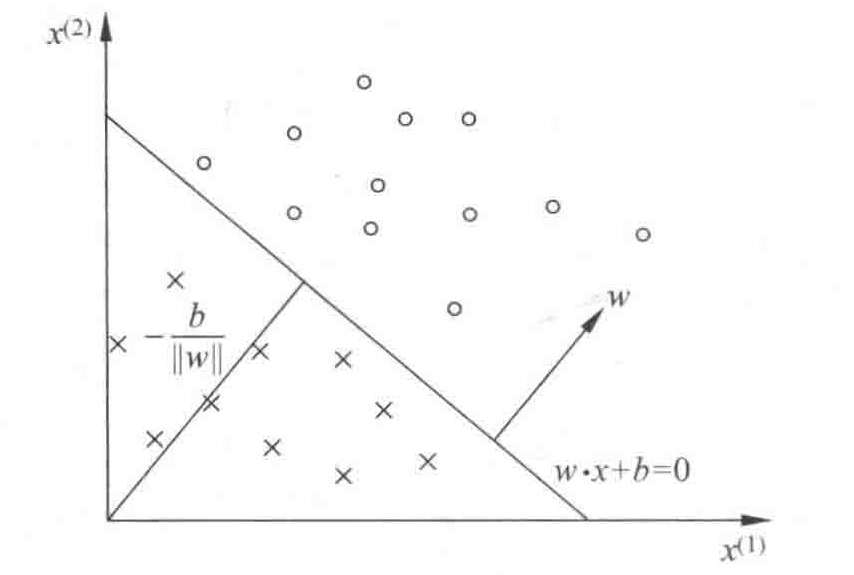# 感知机

## 一、基本形式

感知机是一种线性二分类模型，输入为实例的特征向量，输出为实例的判别，取 $+1,\ -1$ 二值。实际上感知机将输入空间（特征空间）中的实例划分为正负两类的分离超平面。
导入基于误分类的损失函数，利用梯度下降法对损失函数进行极小化，求得感知机模型。

1. 感知机模型
假设输入空间（特征空间）是 $\mathcal{X}\in \mathbf{R}^{\text{n}}$ ，输出空间是 $\mathcal{Y}=\left \{ +1,-1\right \}$ 。输入 $\mathcal{x}\in \mathcal{X}$ 表示实例的特征向量，对应与输入空间的点；输出 $\mathcal{y}\in \mathcal{Y}$ 表示实例的类别。由输入空间到输出空间的如下函数 $$f\left(x\right)=\text{sign}\left(w\cdot x+b\right)$$ 称为感知机。其中 $w$ 和 $b$ 为感知机的模型参数，$w\in \mathbf{R}^{\text{n}}$ 为权值(weight)或权值向量(weight vector)，$b\in \mathbf{R}$ 为偏置(bias)， $w\cdot x$ 表示 $w$ 与 $x$ 的内积。sign是符号函数。

2. 感知机的几何解释
线性方程 $w\cdot x+b=0$ 对应于特征空间 $\mathbf{R}^{\text{n}}$ 中的一个超平面S，其中w是超平面的法向量，b是超平面的截距。此超平面将特征空间划分为两部分，位于两部分的点被分为正负两类，超平面S又叫分离超平面，如图1-1。## 二、感知机的学习策略

感知机学习的目标是求得一个能够将训练集正实例点和负实例点完全正确区分的分离超平面。即确定模型参数 $w,b$ 。

### 2.1 损失函数

感知机的损失函数选择：误分类点到超平面S的总距离。对于输入空间 $\mathbf{R}^{\text{n}}$ 中的任意一点 $x_0$ 到超平面S的距离: $$\frac{1}{\lVert w \rVert}\left| w\cdot x_0+b \right|$$ ，其中 $\lVert w\rVert$ 是 $w$ 的 $L_2$ 范数。
补：$L_2$ 范数: $\lVert w \rVert _2=\left( w_1^2+w_2^2+\cdots +w_n^2 \right) ^{\frac{1}{2}}$ .
其次，对于误分类数据 $\left(x_i,y_i\right)$ ，当 $w\cdot x_i+b>0$ 时， $y_i=-1$ ；而当 $w\cdot x_i+b<0$ 时， $y_i=+1$ 。因此对于任意的误分类点，有$$-y_i\left(w\cdot x_i+b\right)>0$$ 因此，误分类点 $x_i$ 到超平面S的距离是$$-\cfrac{1}{\lVert w \rVert}y_i\left(w\cdot x_i+b\right)$$这样假设超平面S误分类的点的集合为M，那么所有误分类点到超平面S的总距离为$$-\cfrac{1}{\lVert w \rVert}\sum_{x_i\in M}{y_i\left(w\cdot x_i+b\right)}$$不考虑 $\cfrac{1}{\lVert w \rVert}$ ，即得到感知机学习的损失函数。

对于给定的数据集$$T=\left\{\left(x_1,y_1\right) , \left(x_2,y_2\right),\cdots,\left(x_N,y_N\right)\right\}$$ 其中，$x_i\in \mathcal{X}=\mathbf{R}^{\text{n}}$，$y_i\in \mathcal{Y}=\left\{+1,-1\right\}$ , $i=1,2,\cdots, N$。感知机$\text{sign}\left(w\cdot x+b\right)$ 学习的损失函数为$$L\left(w,b\right)=-\sum_{x_i\in M}{y_i\left(w\cdot x_i +b\right)}$$ 其中M为误分类点的集合，损失函数即为感知机学习的经验风险函数。

## 三、感知机学习算法

### 3.1 感知机学习算法的原始形式

首先，任意选取一个超平面$w_0,b_0$，然后用梯度下降法不断极小化目标函数，极小化的过程中不是一次使M中所有误分类点的梯度下降，而是一次随机选取一个误分类点使其梯度下降。
假设误分类点集合M是固定的，那么损失函数$L\left(w,b\right)$的梯度由
$$\bigtriangledown_wL\left( w,b \right) =-\sum_{x_i\in M}{y_ix_i}$$
$$\bigtriangledown_wL\left( w,b \right) =-\sum_{x_i\in M}{y_i}$$

随机选取一个误分类点 $\left(x_i,y_i\right)$ ，对 $w,b$ 进行更新：
$$w\leftarrow w+\eta y_ix_i$$
$$b\leftarrow b+\eta y_i$$

输入：训练数据集 $T=\left\{\left(x_1,y_1\right),\left(x_2,y_2\right),\cdots,\left(x_N,y_N\right)\right\}$ ，其中，$x_i\in \mathcal{X}=\mathbf{R}^{\text{n}}$，$y_i\in \mathcal{Y}=\left\{+1,-1\right\}$ , $i=1,2,\cdots, N$ ；学习率 $\eta \left( 0<\eta \le 1\right)$；
输出：$w,b$ ；感知机模型$f\left(x\right)=\text{sign}\left(w\cdot x+b\right)$。

1. 选取初值 $w_0,b_0$;

2. 在训练集中选取数据 $\left(x_i,y_i\right)$;

3. 如果$y_i\left(w\cdot x_i +b\right)\le 0$，
$$w\leftarrow w+\eta y_ix_i$$
$$b\leftarrow b+\eta y_i$$

4. 转至2，直到训练集中没有误分类点。

Python代码实现如下：

def perceptron(dataArr, labelArr, iter=50):
'''
感知器训练过程
:param dataArr:训练集的数据 (list)
:param labelArr: 训练集的标签(list)
:param iter: 迭代次数，默认50
:return: 训练好的w和b
'''
print('start to trans')
# 将数据转换成矩阵形式（在机器学习中因为通常都是向量的运算，转换称矩阵形式方便运算）
# 转换后的数据中每一个样本的向量都是横向的
dataMat = np.mat(dataArr)
# 将标签转换成矩阵，之后转置(.T为转置)。
# 转置是因为在运算中需要单独取label中的某一个元素，如果是1xN的矩阵的话，无法用label[i]的方式读取
# 对于只有1xN的label可以不转换成矩阵，直接label[i]即可，这里转换是为了格式上的统一
labelMat = np.mat(labelArr).T
# 获取数据矩阵的大小，为m*n
m, n = np.shape(dataMat)
# 创建初始权重w，初始值全为0。
# np.shape(dataMat)的返回值为m，n -> np.shape(dataMat))的值即为n，与
# 样本长度保持一致
w = np.zeros((1, np.shape(dataMat)))
# 初始化偏置b为0
b = 0
# 初始化步长，也就是梯度下降过程中的n，控制梯度下降速率
h = 0.0001

# 进行iter次迭代计算
for k in range(iter):
# 对于每一个样本进行梯度下降
for i in range(m):
# 获取当前样本的向量
xi = dataMat[i]
# 获取当前样本所对应的标签
yi = labelMat[i]
# 判断是否是误分类样本
# 误分类样本特征为： -yi(w*xi+b)>=0
if -1 * yi * (w * xi.T + b) >= 0:
# 对于误分类样本，进行梯度下降，更新w和b
w = w + h * yi * xi
b = b + h * yi
# 打印训练进度
print('Round %d:%d training' % (k, iter))
# 返回训练完的w、b
return w, b

### 3.2 算法的收敛性

对于现行可分数据集，感知机学习算法原始形式收敛，即经过有限次迭代可以得到一个将训练数据集完全划分的分离超平面及感知机模型。
将偏置量 $b$ 并入权重向量 $w$ ，记作 $\hat{w}=\left( w^{\text{T}},b \right) ^{\text{T}}$, 同时扩张输入向量，记作 $\hat{x}=\left(x^{\text{T}},1\right)$。这样，$\hat{x}\in \mathbf{R}^{\text{n+1}}$, $\hat{w}\in \mathbf{R}^{\text{n+1}}$, 显然 $\hat{w}\cdot \hat{x}=w\cdot x+b$.

设训练数据集$T=\left\{\left(x_1,y_1\right),\left(x_2,y_2\right),\cdots,\left(x_N,y_N\right)\right\}$ 是线性可分的，其中$x_i\in \mathcal{X}=\mathbf{R}^{\text{n}}$，$y_i\in \mathcal{Y}=\left\{+1,-1\right\}$ , $i=1,2,\cdots, N$，则

1. 存在满足条件 $\lVert \hat{w}_{\text{opt}}\cdot \hat{x}_i \rVert =1$ 的超平面 $\hat{w}_{\text{opt}}\cdot \hat{x}=w_{\text{opt}}\cdot x+b_{\text{opt}}=0$ 将训练数据集完全正确分开；且存在 $\gamma>0$，对所有$i=1,2,\cdots N$，有$$y_i\left(\hat{w}_{\text{opt}}\cdot \hat{x}_i\right)=y_i\left(w_{\text{opt}}\cdot x+b_{\text{opt}}\right)\ge\gamma$$

2. 令 $R=\underset{1\le i\le N}{\max}\lVert \hat{x}_i \rVert$ ，则感知机算法贼训练数据集上的误分类次数k满足不等式
$$k\le \left (\cfrac{R}{\eta}\right)^2$$

定理表明，误分类次数k是有上界的，经过有限次搜索可以找到将数据集完全正确分开的分离超平面。也就是说，当训练集线性可分时，感知机学习算法原始形式迭代是收敛的。但是，感知机学习算法存在多解，既取决于初值的选择，也依赖于迭代过程中误分类点的选择顺序。

目录